# Does the chromatic symmetric function distinguish between trees?

 Importance: Medium ✭✭
 Author(s): Stanley, Richard P.
 Subject: Graph Theory » Algebraic Graph Theory
 Keywords: chromatic polynomial symmetric function tree
 Posted by: mdevos on: February 25th, 2009
Problem   Do there exist non-isomorphic trees which have the same chromatic symmetric function?

Stanley [S] introduced the following symmetric function associated with a graph. Let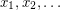be commuting indeterminates, and for every graphlet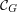be the set of all proper colorings. Then the chromatic symmetric function is defined to be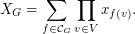So, the coefficient of a termin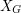is precisely the number of proper colorings ofwhere colorappears exactlytimes. It is immediate thatis homogeneous of degreeand is symmetric.

If we set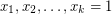andand evaluate, we get the number of proper colorings ofusing the colors. Therefore, the chromatic symmetric function contains all of the information of the chromatic polynomial. In fact, the chromatic symmetric function contains strictly more information about the graph, since there exist examples of graphs which have distinct chromatic symmetric functions but have the same chromatic polynomial.

This natural problem of Stanley remains wide open. It has recently been established for some special classes of trees, namely caterpillars and spiders [MMW].

## Bibliography

[MMW] J. Martin, M. Morin, and J. D. Wagner, On distinguishing trees by their chromatic symmetric functions. J. Combin. Theory Ser. A 115 (2008), no. 2, 237–253. MathSciNet

*[S] R. P. Stanley, A symmetric function generalization of the chromatic polynomial of a graph, Advances in Math. 111 (1995), 166–194.

* indicates original appearance(s) of problem.